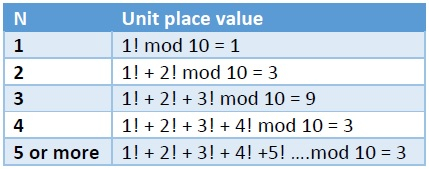# Find the unit place digit of sum of N factorials using C++.

Here we will see how to get the unit place digit of the sum of N factorials. So if N is 3, then after getting sum, we will get 1! + 2! + 3! = 9, this will be the result, for N = 4, it will be 1! + 2! + 3! + 4! = 33. so unit place is 3. If we see this clearly, then as the factorials of N > 5, the unit place is 0, so after 5, it will not contribute to change the unit place. For N = 4 and more, it will be 3. We can make a chart for the unit place, and that will be used in the program.## Example

#include<iostream>
#include<cmath>
using namespace std;
double getUnitPlace(int n) {
int placeVal = {-1, 1, 3, 9, 3};
if(n > 4){
n = 4;
}
return placeVal[n];
}
int main() {
for(int i = 1; i<10; i++){
cout << "Unit place value of sum of factorials when N = "<<i<<" is: " << getUnitPlace(i) << endl;
}
}

## Output

Unit place value of sum of factorials when N = 1 is: 1
Unit place value of sum of factorials when N = 2 is: 3
Unit place value of sum of factorials when N = 3 is: 9
Unit place value of sum of factorials when N = 4 is: 3
Unit place value of sum of factorials when N = 5 is: 3
Unit place value of sum of factorials when N = 6 is: 3
Unit place value of sum of factorials when N = 7 is: 3
Unit place value of sum of factorials when N = 8 is: 3
Unit place value of sum of factorials when N = 9 is: 3

Updated on: 30-Oct-2019

71 Views